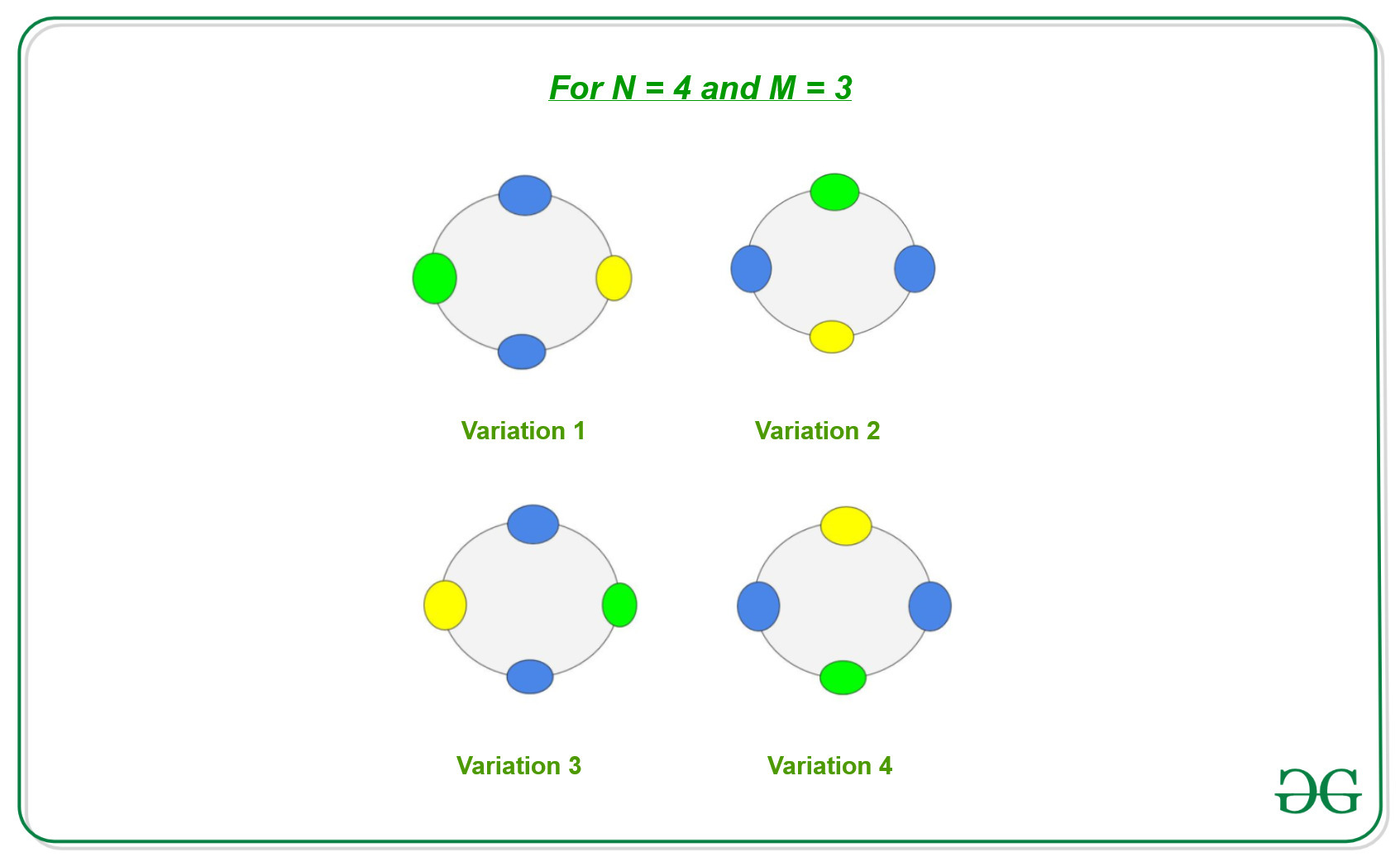# Orbit counting theorem or Burnside’s Lemma

Burnside’s Lemma is also sometimes known as orbit counting theorem. It is one of the results of group theory. It is used to count distinct objects with respect to symmetry. It basically gives us the formula to count the total number of combinations, where two objects that are symmetrical to each other with respect to rotation or reflection are counted as a single representative.

Therefore, Burnside Lemma’s states that total number of distinct object is:where:

• c(k) is the number of combination that remains unchanged when Kth rotation is applied, and
• N is the total number of ways to change the position of N elements.

For Example:

Let us consider we have a necklace of N stones and we can color it with M colors. If two necklaces are similar after rotation then the two necklaces are considered to be similar and counted as one different combination. Now Let’s suppose we have N = 4 stones with M = 3 colors, then

Since we have N stones, therefore, we have N possible variations of each necklace by rotation:Observations:

1. There are N ways to change the position of necklace as we can rotate it by 0 to N – 1 times.
2. There areways to color a necklace. If the number of rotation is 0, then allways remains different.
3. If the number of rotation is 1, then there in only M necklaces which will be different out of allways.
4. Generally if the number of rotation is K,necklaces will remain sames out of allways.

Therefore for total number of distinct necklaces of N stones after coloring with M colors is the summation of all the distinct necklaces at each rotation. It is given by:Below is the implementation of the above approach:

## C++

 // C++ program for implementing the  // Orbit counting theorem  // or Burnside's Lemma     #include  using namespace std;     // Function to find result using  // Orbit counting theorem  // or Burnside's Lemma  void countDistinctWays(int N, int M)  {         int ans = 0;         // According to Burnside's Lemma      // calculate distinct ways for each      // rotation      for (int i = 0; i < N; i++) {             // Find GCD          int K = __gcd(i, N);          ans += pow(M, K);      }         // Divide By N      ans /= N;         // Print the distinct ways      cout << ans << endl;  }     // Driver Code  int main()  {         // N stones and M colors      int N = 4, M = 3;         // Function call      countDistinctWays(N, M);         return 0;  }

Output:

24


Attention reader! Don’t stop learning now. Get hold of all the important DSA concepts with the DSA Self Paced Course at a student-friendly price and become industry ready.

My Personal Notes arrow_drop_upCheck out this Author's contributed articles.

If you like GeeksforGeeks and would like to contribute, you can also write an article using contribute.geeksforgeeks.org or mail your article to contribute@geeksforgeeks.org. See your article appearing on the GeeksforGeeks main page and help other Geeks.

Please Improve this article if you find anything incorrect by clicking on the "Improve Article" button below.